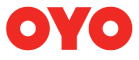New update is available. Click here to update.
Topics

# Chocolate Fest

Moderate0/80
Average time to solve is 25m## Problem statement

Alex is taking part in a chocolate eating competition. There are ‘N’ boxes of chocolate numbered ‘0’ to ‘N - 1’. Each box contains some chocolates. The boxes are arranged in a line, with the box ‘0’ being the nearest to Alex. To win, Alex has to eat more than ‘X’ number of chocolates using minimum boxes. Alex can only eat chocolates from contiguous boxes. That is, he can choose some i and j (i <= j) and eat all the chocolates from box i, box i + 1, ..., box j. Alex is lazy, so if there are many optimal choices, he will choose the boxes nearest to him.

Given an array ‘choco’ containing the number of chocolates in each box, predict the boxes that Alex will choose. It is guaranteed that one such choice always exists.

Detailed explanation ( Input/output format, Notes, Images )
Constraints:
``````1 <= T <= 5
1 <= N <= 10^5
1 <= X <= 10^9
1<= choco[i] <= 10^4

Where ‘T’ is the number of test cases, ‘N’ is the number of boxes, ‘X’ is the target, and ‘choco[i]’ is the number of chocolates in the box ‘i’, where 0 <= i <= N - 1.

Time Limit: 1 sec
``````
Sample Input 1:
``````2
6 9
1 3 5 6 4 3
3 10
11 2 5
``````
Sample Output 1:
``````5 6
11
``````
Explanation for Sample Input 1:
``````In the first test case, the number of chocolates is [1, 3, 5, 6, 4, 3]. We can see, Alex can eat more than 9 chocolates using a minimum of two boxes. Alex can use more boxes, but according to the question, he must use minimum boxes. So, he has the choice of picking boxes (2,3), (3,4). But since box two is nearest to him, he chooses box 2 and box 3. Thus the result is the chocolates in these boxes, this is, 5 and 6.

In the second test case, the number of chocolates is [11, 2, 5]. So Alex can eat more than 10 chocolates using one box only. He picks box 0. So the answer is 11.
``````
Sample Input 2:
``````2
5 12
5 7 11 3 5
3 20
20 19 21
``````
Sample Output 2:
``````7 11
21
``````Console